Definition: division from The Penguin Dictionary of Science

The inverse operation to multiplication: finding that number (the quotient) which, when multiplied by one given number (the divisor), yields a second given number (the dividend). With dividend a and divisor b the quotient is denoted a/b.

Summary Article: Lesson 1-6: Division
From Homework Helpers: Basic Math and Pre-Algebra

Division is the reverse, or undoing, of multiplication. Division is related to multiplication in the same way that subtraction is related to addition. Because 4 × 5 = 20 we say thatand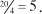Being familiar with the multiplication table will be very helpful when solving division problems.

The symbols used for division are ÷, −, /, or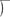. The expressions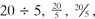and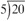all mean the same thing. The number 20 in all of the examples is the number that gets divided; it is called the dividend. The number 5 in all of the examples is the number that divides into the dividend; it is called the divisor. The answer obtained after doing the division is called the quotient. Just as with subtraction, order matters; division is not commutative.

To work out a division problem involving two-digit numbers, it is best to use long division. I will review long division in the first example.

Example 1

Divide: 348 ÷ 5

Solution: Write the problem as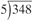. Divide the divisor (in this case, 5) into the left digit (or digits) of the dividend (in this case, 348). To do this, choose the smallest part of the dividend that the divisor will divide in to. Because 5 is greater than 3, we need to use the first two digits of our dividend: 34. Look for the largest multiple of 5 that is less than 34. Because 5 × 6 = 30 and 5 × 7 = 35, the largest multiple of 5 that is less than 34 is 30. Place a 6 (the other factor involved in the product that gives 30) above the 4 (the last digit of the dividend under consideration), put 30 underneath the 34 and subtract 30 from 34, and bring down the 8 from the original 348: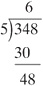Now do the same thing with the next two digits. Look for the largest multiple of 5 that is less than 48. Because 5 × 9 = 45, that's what we need. Write a 9 next to the 6 and 45 underneath the 48, and subtract: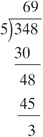Because 3 is less than 5, and there are no more digits to the right to incorporate, we are done. The number 3 in this example is called the remainder. The remainder is the number “left over” when we are finished doing the long division. So 348 ÷ 5 is equal to 69 remainder 3.

A smaller number is a factor of a larger number if, when you divide the smaller number into the larger number you end up with a remainder of 0. It is called a factor because you can multiply it by the quotient to get the larger number. Remember that multiplication and division go hand-in-hand. For example, 4 is a factor of 20 because 20 ÷ 4 equals 5 remainder 0. Looking at it another way, 4 × 5 = 20. We say that 20 is evenly divisible by 4. Every number is evenly divisible by its factors. “Divides evenly” means the same thing as “has remainder 0.” Every whole number is evenly divisible by 1, and every non-zero whole number is evenly divisible by itself.Copyright 2011 © by Denise Szecsei

### Related Articles

##### Full text Article division
The Penguin Dictionary of Mathematics

The inverse operation to multiplication; the operation of finding-for two numbers or polynomials -a third number or polynomial (the quotient )...

##### Full text Article division
The Penguin English Dictionary

/divizh(ə)n/ noun 1 the action of dividing or process of being divided. 2 a the action of dividing or process of being...

##### Full text Article divide 1
Merriam-Webster's Collegiate(R) Dictionary

pronunciation vt (14c) 1 a : to separate into two or more parts, areas, or groups 〈⁓ the city into wards〉 b : to separate into classes, categories,

See more from Credo